Technology

# Getting Started with TensorFlow: A Machine Learning Tutorial

Dino (BCS) has 6+ years in software development, specializing in back-end and security work using Java, Elasticsearch, .NET, and Python.

TensorFlow is an open source software library created by Google that is used to implement machine learning and deep learning systems. These two names contain a series of powerful algorithms that share a common challenge—to allow a computer to learn how to automatically spot complex patterns and/or to make best possible decisions.

If you’re interested in details about these systems, you can learn more from the Toptal blog posts on machine learning and deep learning.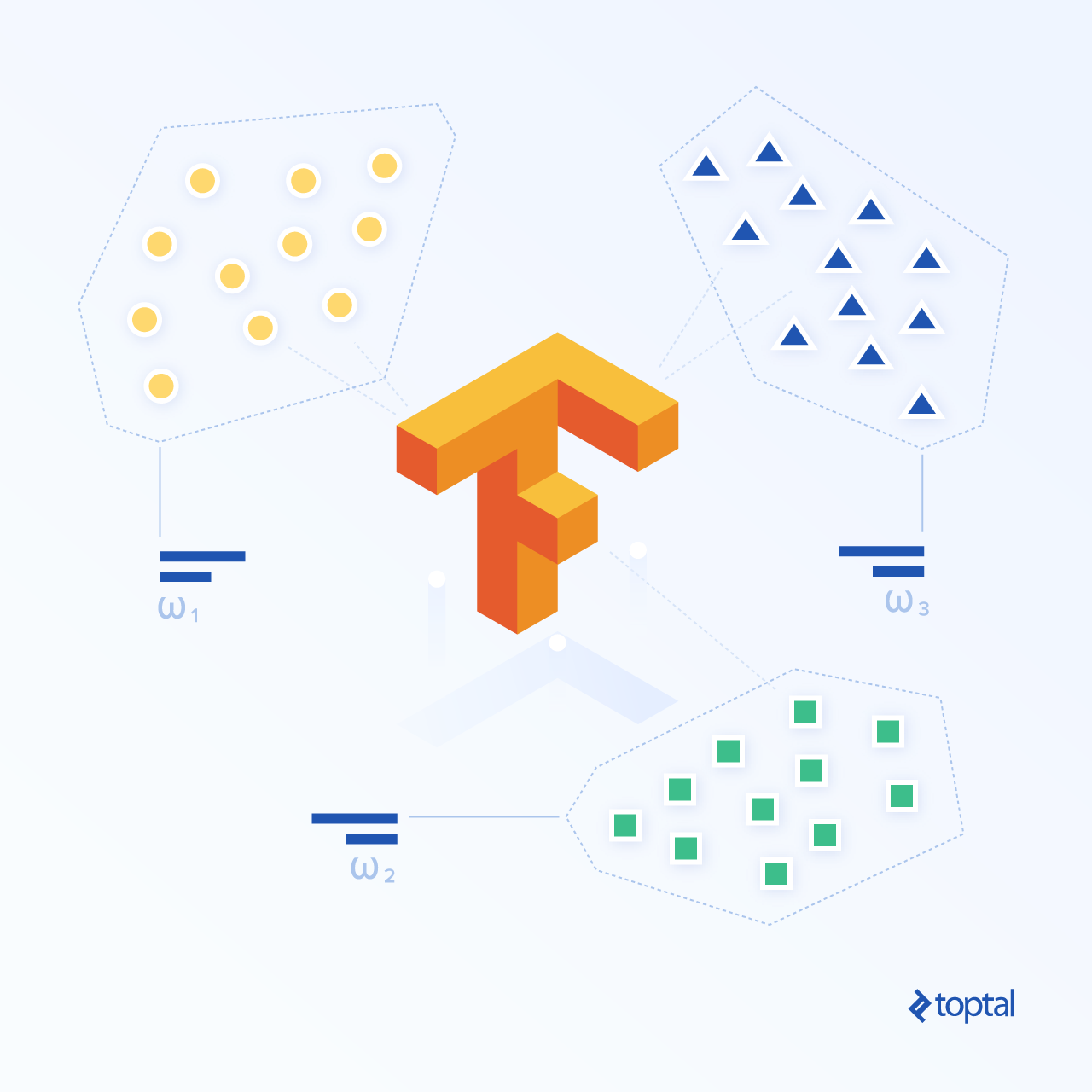TensorFlow, at its heart, is a library for dataflow programming. It leverages various optimization techniques to make the calculation of mathematical expressions easier and more performant.

Some of the key features of TensorFlow are:

• Efficiently works with mathematical expressions involving multi-dimensional arrays
• Good support of deep neural networks and machine learning concepts
• GPU/CPU computing where the same code can be executed on both architectures
• High scalability of computation across machines and huge data sets

Together, these features make TensorFlow the perfect framework for machine intelligence at a production scale.

In this TensorFlow tutorial, you will learn how you can use simple yet powerful machine learning methods in TensorFlow and how you can use some of its auxiliary libraries to debug, visualize, and tweak the models created with it.

## Installing TensorFlow

We will be using the TensorFlow Python API, which works with Python 2.7 and Python 3.3+. The GPU version (Linux only) requires the Cuda Toolkit 7.0+ and cuDNN v2+.

We shall use the Conda package dependency management system to install TensorFlow. Conda allows us to separate multiple environments on a machine. You can learn how to install Conda from here.

After installing Conda, we can create the environment that we will use for TensorFlow installation and use. The following command will create our environment with some additional libraries like NumPy, which is very useful once we start to use TensorFlow.

The Python version installed inside this environment is 2.7, and we will use this version in this article.

``````conda create --name TensorflowEnv biopython
``````

To make things easy, we are installing biopython here instead of just NumPy. This includes NumPy and a few other packages that we will be needing. You can always install the packages as you need them using the `conda install` or the `pip install` commands.

The following command will activate the created Conda environment. We will be able to use packages installed within it, without mixing with packages that are installed globally or in some other environments.

``````source activate TensorFlowEnv
``````

The pip installation tool is a standard part of a Conda environment. We will use it to install the TensorFlow library. Prior to doing that, a good first step is updating pip to the latest version, using the following command:

``````pip install --upgrade pip
``````

Now we are ready to install TensorFlow, by running:

``````pip install tensorflow
``````

The download and build of TensorFlow can take several minutes. At the time of writing, this installs TensorFlow 1.1.0.

## Data Flow Graphs

In TensorFlow, computation is described using data flow graphs. Each node of the graph represents an instance of a mathematical operation (like addition, division, or multiplication) and each edge is a multi-dimensional data set (tensor) on which the operations are performed.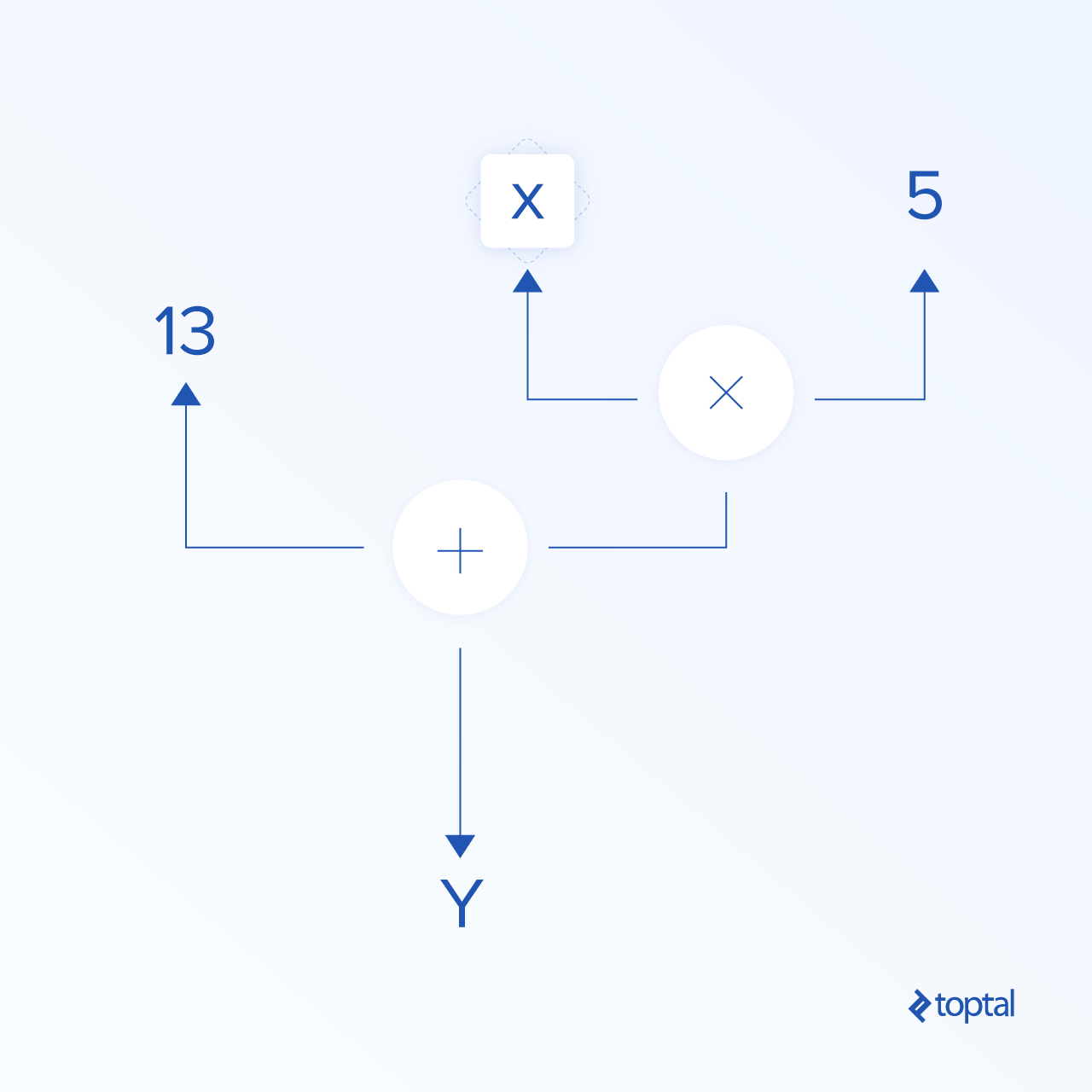As TensorFlow works with computational graphs, they are managed where each node represents the instantiation of an operation where each operation has zero or more inputs and zero or more outputs.

Edges in TensorFlow can be grouped in two categories: Normal edges transfer data structure (tensors) where it is possible that the output of one operation becomes the input for another operation and special edges, which are used to control dependency between two nodes to set the order of operation where one node waits for another to finish.

### Simple Expressions

Before we move on to discuss elements of TensorFlow, we will first do a session of working with TensorFlow, to get a feeling of what a TensorFlow program looks like.

Let’s start with simple expressions and assume that, for some reason, we want to evaluate the function `y = 5*x + 13` in TensorFlow fashion.

In simple Python code, it would look like:

``````x = -2.0
y = 5*x + 13
print y
``````

which gives us in this case a result of 3.0.

Now we will convert the above expression into TensorFlow terms.

#### Constants

In TensorFlow, constants are created using the function constant, which has the signature `constant(value, dtype=None, shape=None, name='Const', verify_shape=False)`, where `value` is an actual constant value which will be used in further computation, `dtype` is the data type parameter (e.g., float32/64, int8/16, etc.), `shape` is optional dimensions, `name` is an optional name for the tensor, and the last parameter is a boolean which indicates verification of the shape of values.

If you need constants with specific values inside your training model, then the `constant` object can be used as in following example:

``````z = tf.constant(5.2, name="x", dtype=tf.float32)
``````

#### Variables

Variables in TensorFlow are in-memory buffers containing tensors which have to be explicitly initialized and used in-graph to maintain state across session. By simply calling the constructor the variable is added in computational graph.

Variables are especially useful once you start with training models, and they are used to hold and update parameters. An initial value passed as an argument of a constructor represents a tensor or object which can be converted or returned as a tensor. That means if we want to fill a variable with some predefined or random values to be used afterwards in the training process and updated over iterations, we can define it in the following way:

``````k = tf.Variable(tf.zeros(), name="k")
``````

Another way to use variables in TensorFlow is in calculations where that variable isn’t trainable and can be defined in the following way:

``````k = tf.Variable(tf.add(a, b), trainable=False)
``````

#### Sessions

In order to actually evaluate the nodes, we must run a computational graph within a session.

A session encapsulates the control and state of the TensorFlow runtime. A session without parameters will use the default graph created in the current session, otherwise the session class accepts a graph parameter, which is used in that session to be executed.

Below is a brief code snippet that shows how the terms defined above can be used in TensorFlow to calculate a simple linear function.

``````import tensorflow as tf

x = tf.constant(-2.0, name="x", dtype=tf.float32)
a = tf.constant(5.0, name="a", dtype=tf.float32)
b = tf.constant(13.0, name="b", dtype=tf.float32)

init = tf.global_variables_initializer()

with tf.Session() as session:
session.run(init)
print session.run(y)
``````

## Using TensorFlow: Defining Computational Graphs

The good thing about working with dataflow graphs is that the execution model is separated from its execution (on CPU, GPU, or some combination) where, once implemented, software in TensorFlow can be used on the CPU or GPU where all complexity related to code execution is hidden.

The computation graph can be built in the process of using the TensorFlow library without having to explicitly instantiate Graph objects.

A Graph object in TensorFlow can be created as a result of a simple line of code like `c = tf.add(a, b)`. This will create an operation node that takes two tensors `a` and `b` that produce their sum `c` as output.

The computation graph is a built-in process that uses the library without needing to call the graph object directly. A graph object in TensorFlow, which contains a set of operations and tensors as units of data, is used between operations which allows the same process and contains more than one graph where each graph will be assigned to a different session. For example, the simple line of code `c = tf.add(a, b)` will create an operation node that takes two tensors `a` and `b` as input and produces their sum `c` as output.

TensorFlow also provides a feed mechanism for patching a tensor to any operation in the graph, where the feed replaces the output of an operation with the tensor value. The feed data are passed as an argument in the `run()` function call.

A placeholder is TensorFlow’s way of allowing developers to inject data into the computation graph through placeholders which are bound inside some expressions. The signature of the placeholder is:

``````placeholder(dtype, shape=None, name=None)
``````

where dtype is the type of elements in the tensors and can provide both the shape of the tensors to be fed and the name for the operation.

If the shape isn’t passed, this tensor can be fed with any shape. An important note is that the placeholder tensor has to be fed with data, otherwise, upon execution of the session and if that part is missing, the placeholder generates an error with the following structure:

``````InvalidArgumentError (see above for traceback): You must feed a value for placeholder tensor 'y' with dtype float
``````

The advantage of placeholders is that they allow developers to create operations, and the computational graph in general, without needing to provide the data in advance for that, and the data can be added in runtime from external sources.

Let’s take a simple problem of multiplying two integers `x` and `y` in TensorFlow fashion, where a placeholder will be used together with a feed mechanism through the session `run` method.

``````import tensorflow as tf

x = tf.placeholder(tf.float32, name="x")
y = tf.placeholder(tf.float32, name="y")

z = tf.multiply(x, y, name="z")

with tf.Session() as session:
print session.run(z, feed_dict={x: 2.1, y: 3.0})
``````

## Visualizing the Computational Graph with TensorBoard

TensorBoard is a visualization tool for analyzing data flow graphs. This can be useful for gaining better understanding of machine learning models.

With TensorBoard, you can gain insight into different types of statistics about the parameters and details about the parts of the computational graph in general. It is not unusual that a deep neural network has large number of nodes. TensorBoard allows developers to get insight into each node and how the computation is executed over the TensorFlow runtime.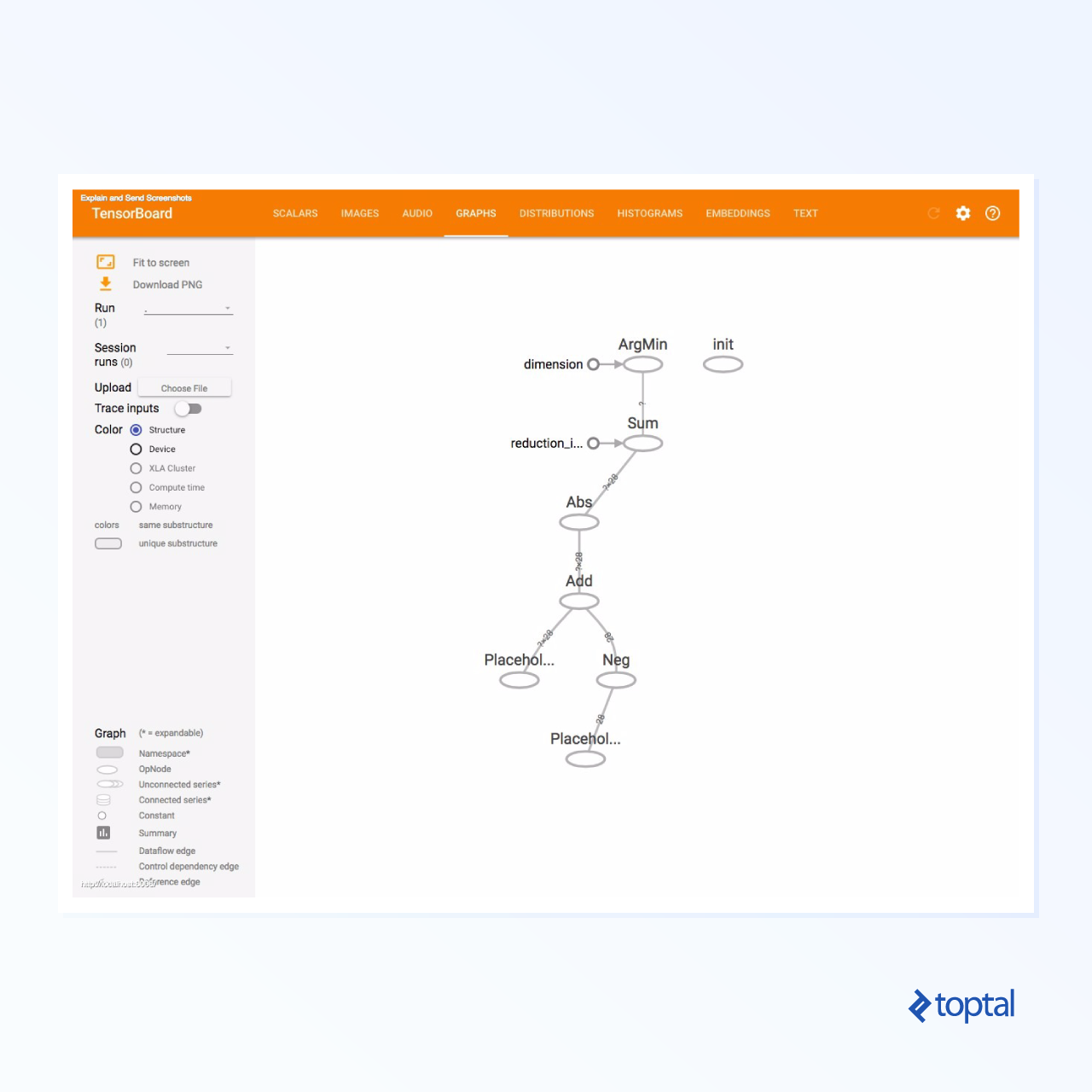Now let’s get back to our example from the beginning of this TensorFlow tutorial where we defined a linear function with the format `y = a*x + b`.

In order to log events from session which later can be used in TensorBoard, TensorFlow provides the `FileWriter` class. It can be used to create an event file for storing summaries and events where the constructor accepts six parameters and looks like:

``````__init__(logdir, graph=None, max_queue=10, flush_secs=120, graph_def=None, filename_suffix=None)
``````

where the logdir parameter is required, and others have default values. The graph parameter will be passed from the session object created in the training program. The full example code looks like:

``````import tensorflow as tf

x = tf.constant(-2.0, name="x", dtype=tf.float32)
a = tf.constant(5.0, name="a", dtype=tf.float32)
b = tf.constant(13.0, name="b", dtype=tf.float32)

init = tf.global_variables_initializer()

with tf.Session() as session:
merged = tf.summary.merge_all() // new
writer = tf.summary.FileWriter("logs", session.graph) // new

session.run(init)
print session.run(y)
``````

We added just two new lines. We merge all the summaries collected in the default graph, and `FileWriter` is used to dump events to the file as we described above, respectively.

After running the program, we have the file in the directory logs, and the last step is to run `tensorboard`:

``````tensorboard --logdir logs/
``````

Now TensorBoard is started and running on the default port 6006. After opening `http://localhost:6006` and clicking on the Graphs menu item (located at the top of the page), you will be able to see the graph, like the one in the picture below: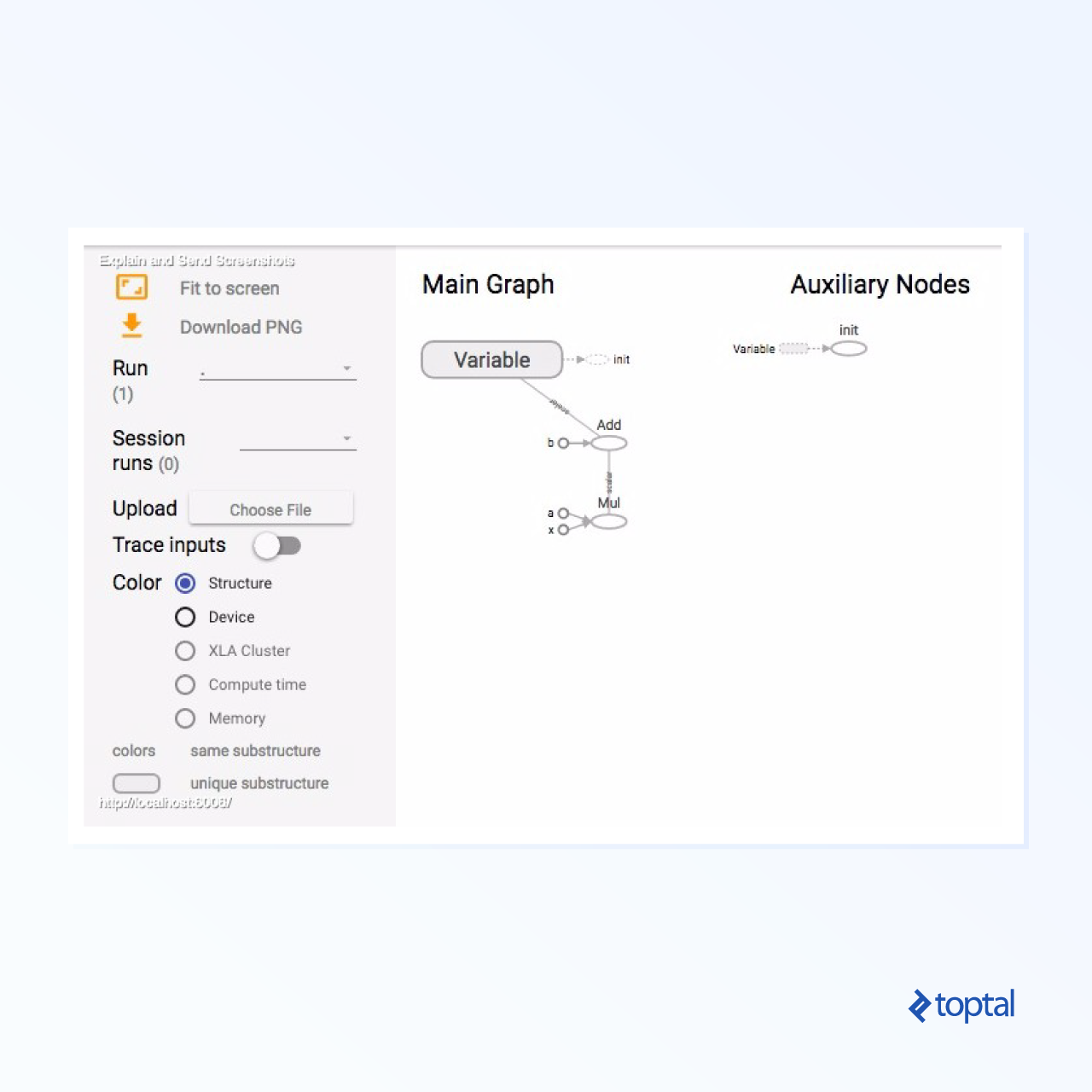TensorBoard marks constants and summary nodes specific symbols, which are described below.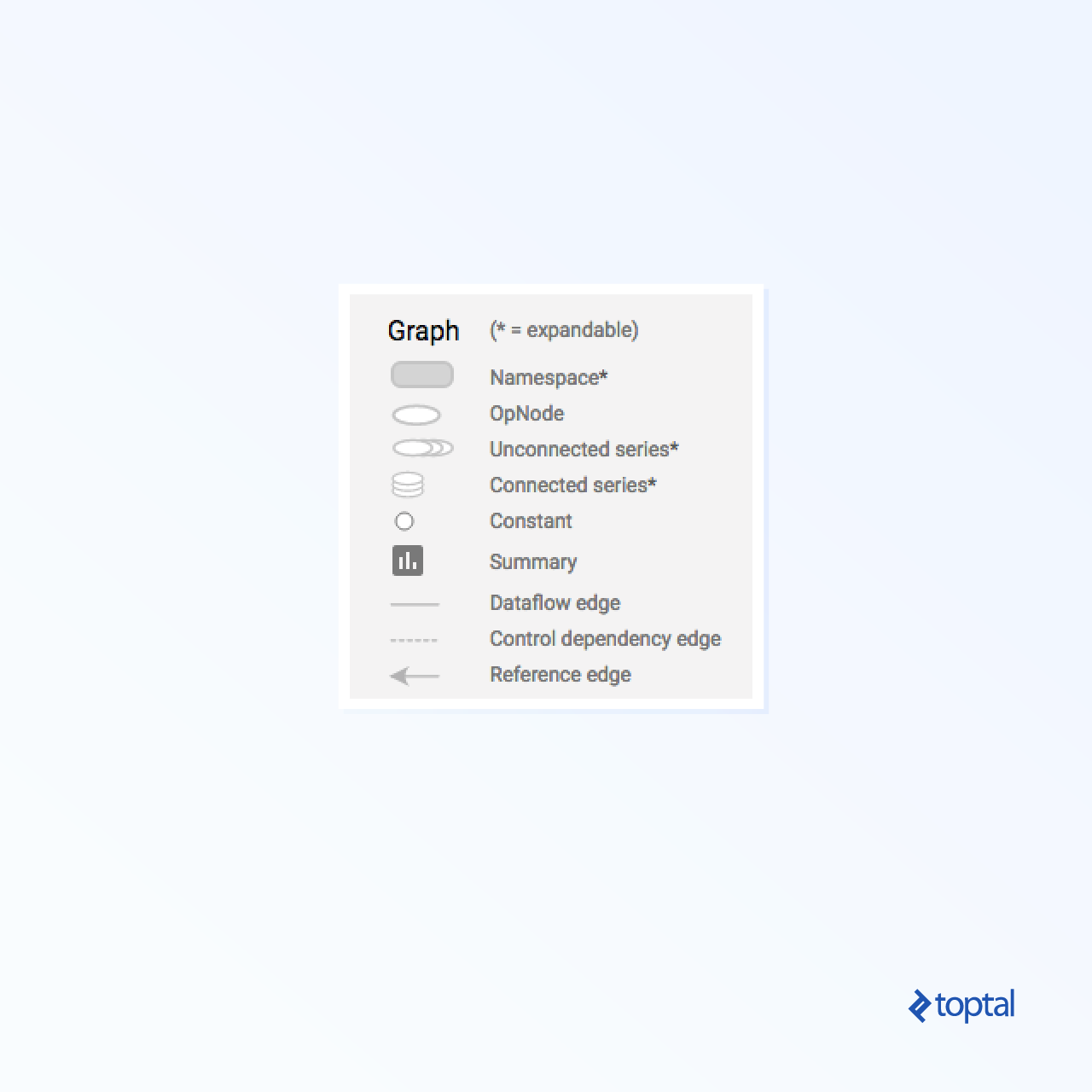## Mathematics with TensorFlow

Tensors are the basic data structures in TensorFlow, and they represent the connecting edges in a dataflow graph.

A tensor simply identifies a multidimensional array or list. The tensor structure can be identified with three parameters: rank, shape, and type.

• Rank: Identifies the number of dimensions of the tensor. A rank is known as the order or n-dimensions of a tensor, where for example rank 1 tensor is a vector or rank 2 tensor is matrix.
• Shape: The shape of a tensor is the number of rows and columns it has.
• Type: The data type assigned to tensor elements.

To build a tensor in TensorFlow, we can build an n-dimensional array. This can be done easily by using the NumPy library, or by converting a Python n-dimensional array into a TensorFlow tensor.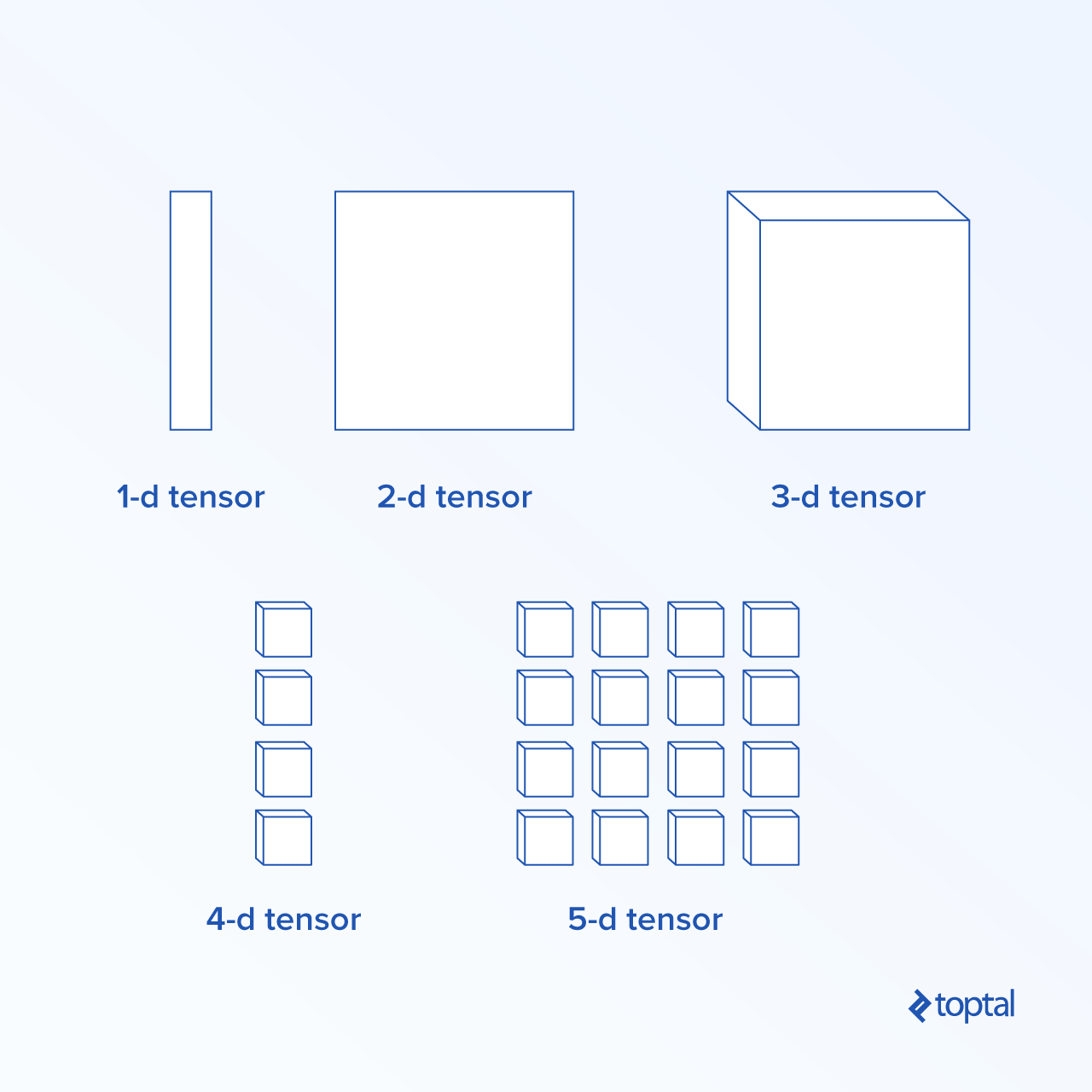To build a 1-d tensor, we will use a NumPy array, which we’ll construct by passing a built-in Python list.

``````import numpy as np
tensor_1d = np.array([1.45, -1, 0.2, 102.1])
``````

Working with this kind of array is similar to working with a built-in Python list. The main difference is that the NumPy array also contains some additional properties, like dimension, shape, and type.

``````> > print tensor1d
[   1.45   -1.      0.2   102.1 ]

> > print tensor1d
1.45

> > print tensor1d
0.2

> > print tensor1d.ndim
1

> > print tensor1d.shape
(4,)

> > print tensor1d.dtype
float64
``````

A NumPy array can be easily converted into a TensorFlow tensor with the auxiliary function convert_to_tensor, which helps developers convert Python objects to tensor objects. This function accepts tensor objects, NumPy arrays, Python lists, and Python scalars.

``````tensor = tf.convert_to_tensor(tensor_1d, dtype=tf.float64)
``````

Now if we bind our tensor to the TensorFlow session, we will be able to see the results of our conversion.

``````tensor = tf.convert_to_tensor(tensor_1d, dtype=tf.float64)

with tf.Session() as session:
print session.run(tensor)
print session.run(tensor)
print session.run(tensor)
``````

Output:

``````[   1.45   -1.      0.2   102.1 ]
1.45
-1.0
``````

We can create a 2-d tensor, or matrix, in a similar way:

``````tensor_2d = np.array(np.random.rand(4, 4), dtype='float32')
tensor_2d_1 = np.array(np.random.rand(4, 4), dtype='float32')
tensor_2d_2 = np.array(np.random.rand(4, 4), dtype='float32')

m1 = tf.convert_to_tensor(tensor_2d)
m2 = tf.convert_to_tensor(tensor_2d_1)
m3 = tf.convert_to_tensor(tensor_2d_2)
mat_product = tf.matmul(m1, m2)
mat_det = tf.matrix_determinant(m3)

with tf.Session() as session:
print session.run(mat_product)
print session.run(mat_sum)
print session.run(mat_det)
``````

### Tensor Operations

In the example above, we introduce a few TensorFlow operations on the vectors and matrices. The operations perform certain calculations on the tensors. Which calculations those are is shown in the table below.

TensorFlow operator Description
tf.subtract x-y
tf.multiply x*y
tf.div x/y
tf.mod x % y
tf.abs |x|
tf.negative -x
tf.sign sign(x)
tf.square x*x
tf.round round(x)
tf.sqrt sqrt(x)
tf.pow x^y
tf.exp e^x
tf.log log(x)
tf.maximum max(x, y)
tf.minimum min(x, y)
tf.cos cos(x)
tf.sin sin(x)

TensorFlow operations listed in the table above work with tensor objects, and are performed element-wise. So if you want to calculate the cosine for a vector x, the TensorFlow operation will do calculations for each element in the passed tensor.

``````tensor_1d = np.array([0, 0, 0])
tensor = tf.convert_to_tensor(tensor_1d, dtype=tf.float64)
with tf.Session() as session:
print session.run(tf.cos(tensor))
``````

Output:

``````[ 1.  1.  1.]
``````

### Matrix Operations

Matrix operations are very important for machine learning models, like linear regression, as they are often used in them. TensorFlow supports all the most common matrix operations, like multiplication, transposing, inversion, calculating the determinant, solving linear equations, and many more.

Next up, we will explain some of the matrix operations. They tend to be important when comes to machine learning models, like in linear regression. Let’s write some code that will do basic matrix operations like multiplication, getting the transpose, getting the determinant, multiplication, sol, and many more.

Below are basic examples of calling these operations.

``````import tensorflow as tf
import numpy as np

def convert(v, t=tf.float32):
return tf.convert_to_tensor(v, dtype=t)

m1 = convert(np.array(np.random.rand(4, 4), dtype='float32'))
m2 = convert(np.array(np.random.rand(4, 4), dtype='float32'))
m3 = convert(np.array(np.random.rand(4, 4), dtype='float32'))
m4 = convert(np.array(np.random.rand(4, 4), dtype='float32'))
m5 = convert(np.array(np.random.rand(4, 4), dtype='float32'))

m_tranpose = tf.transpose(m1)
m_mul = tf.matmul(m1, m2)
m_det = tf.matrix_determinant(m3)
m_inv = tf.matrix_inverse(m4)
m_solve = tf.matrix_solve(m5, [, , , ])

with tf.Session() as session:
print session.run(m_tranpose)
print session.run(m_mul)
print session.run(m_inv)
print session.run(m_det)
print session.run(m_solve)
``````

### Transforming Data

#### Reduction

TensorFlow supports different kinds of reduction. Reduction is an operation that removes one or more dimensions from a tensor by performing certain operations across those dimensions. A list of supported reductions for the current version of TensorFlow can be found here. We will present a few of them in the example below.

``````import tensorflow as tf
import numpy as np

def convert(v, t=tf.float32):
return tf.convert_to_tensor(v, dtype=t)

x = convert(
np.array(
[
(1, 2, 3),
(4, 5, 6),
(7, 8, 9)
]), tf.int32)

bool_tensor = convert([(True, False, True), (False, False, True), (True, False, False)], tf.bool)

red_sum_0 = tf.reduce_sum(x)
red_sum = tf.reduce_sum(x, axis=1)

red_prod_0 = tf.reduce_prod(x)
red_prod = tf.reduce_prod(x, axis=1)

red_min_0 = tf.reduce_min(x)
red_min = tf.reduce_min(x, axis=1)

red_max_0 = tf.reduce_max(x)
red_max = tf.reduce_max(x, axis=1)

red_mean_0 = tf.reduce_mean(x)
red_mean = tf.reduce_mean(x, axis=1)

red_bool_all_0 = tf.reduce_all(bool_tensor)
red_bool_all = tf.reduce_all(bool_tensor, axis=1)

red_bool_any_0 = tf.reduce_any(bool_tensor)
red_bool_any = tf.reduce_any(bool_tensor, axis=1)

with tf.Session() as session:
print "Reduce sum without passed axis parameter: ", session.run(red_sum_0)
print "Reduce sum with passed axis=1: ", session.run(red_sum)

print "Reduce product without passed axis parameter: ", session.run(red_prod_0)
print "Reduce product with passed axis=1: ", session.run(red_prod)

print "Reduce min without passed axis parameter: ", session.run(red_min_0)
print "Reduce min with passed axis=1: ", session.run(red_min)

print "Reduce max without passed axis parameter: ", session.run(red_max_0)
print "Reduce max with passed axis=1: ", session.run(red_max)

print "Reduce mean without passed axis parameter: ", session.run(red_mean_0)
print "Reduce mean with passed axis=1: ", session.run(red_mean)

print "Reduce bool all without passed axis parameter: ", session.run(red_bool_all_0)
print "Reduce bool all with passed axis=1: ", session.run(red_bool_all)

print "Reduce bool any without passed axis parameter: ", session.run(red_bool_any_0)
print "Reduce bool any with passed axis=1: ", session.run(red_bool_any)
``````

Output:

``````Reduce sum without passed axis parameter:  45
Reduce sum with passed axis=1:  [ 6 15 24]
Reduce product without passed axis parameter:  362880
Reduce product with passed axis=1:  [  6 120 504]
Reduce min without passed axis parameter:  1
Reduce min with passed axis=1:  [1 4 7]
Reduce max without passed axis parameter:  9
Reduce max with passed axis=1:  [3 6 9]
Reduce mean without passed axis parameter:  5
Reduce mean with passed axis=1:  [2 5 8]
Reduce bool all without passed axis parameter:  False
Reduce bool all with passed axis=1:  [False False False]
Reduce bool any without passed axis parameter:  True
Reduce bool any with passed axis=1:  [ True  True  True]
``````

The first parameter of reduction operators is the tensor that we want to reduce. The second parameter is the indexes of dimensions along which we want to perform the reduction. That parameter is optional, and if not passed, reduction will be performed along all dimensions.

We can take a look at the reduce_sum operation. We pass a 2-d tensor, and want to reduce it along dimension 1.

In our case, the resulting sum would be:

``````[1 + 2 + 3 = 6, 4 + 5 + 6 = 15, 7 + 8 + 9 = 24]
``````

If we passed dimension 0, the result would be:

``````[1 + 4 + 7 = 12, 2 + 5 + 8 = 15, 3 + 6 + 9 = 18]
``````

If we don’t pass any axis, the result is just the overall sum of:

``````1 + 4 + 7 = 12, 2 + 5 + 8 = 15, 3 + 6 + 9 = 45
``````

All reduction functions have a similar interface and are listed in the TensorFlow reduction documentation.

#### Segmentation

Segmentation is a process in which one of the dimensions is the process of mapping dimensions onto provided segment indexes, and the resulting elements are determined by an index row.

Segmentation is actually grouping the elements under repeated indexes, so for example, in our case, we have segmented ids `[0, 0, 1, 2, 2]` applied on tensor `tens1`, meaning that the first and second arrays will be transformed following segmentation operation (in our case summation) and will get a new array, which looks like `(2, 8, 1, 0) = (2+0, 5+3, 3-2, -5+5)`. The third element in tensor `tens1` is untouched because it isn’t grouped in any repeated index, and last two arrays are summed in same way as it was the case for the first group. Beside summation, TensorFlow supports product, mean, max, and min.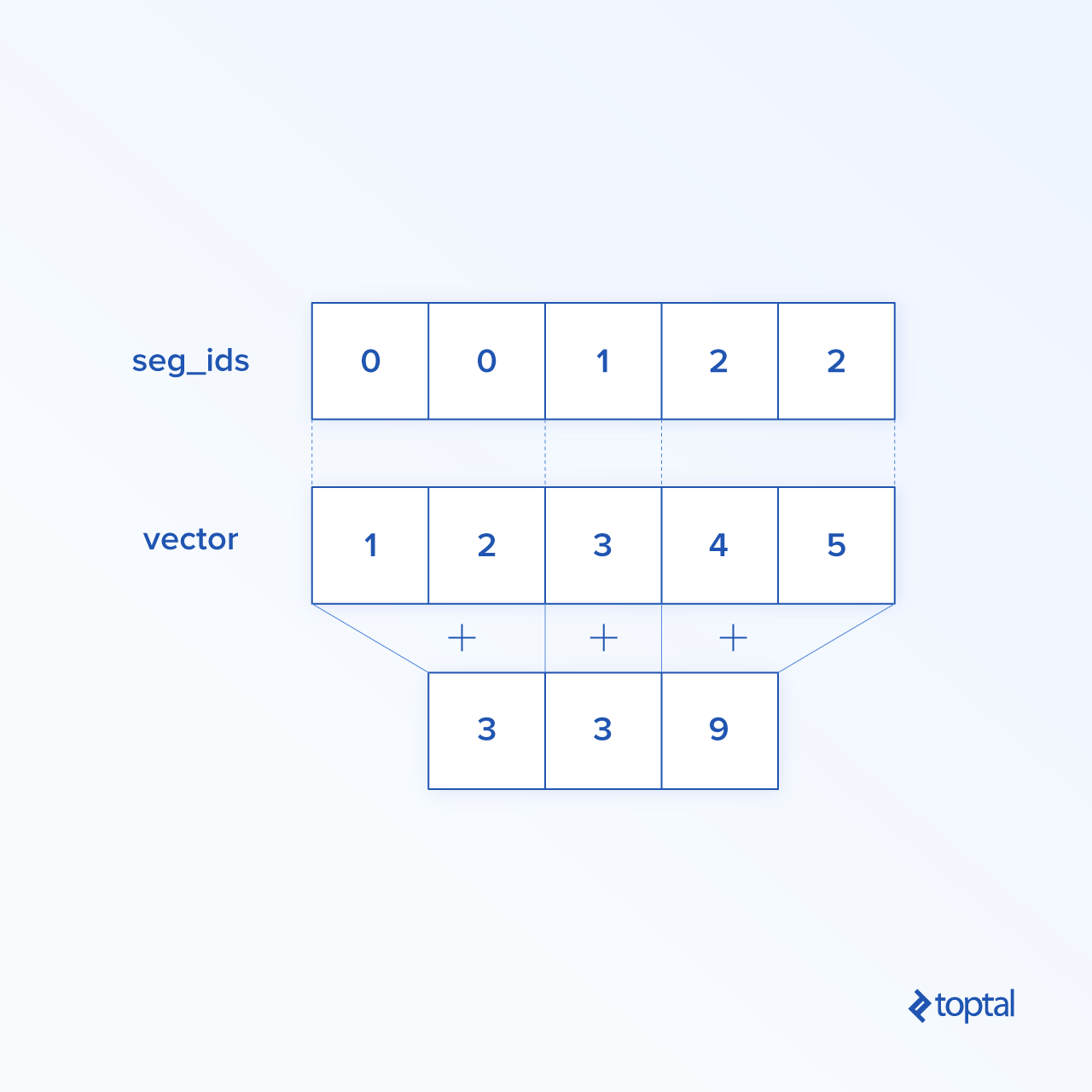``````import tensorflow as tf
import numpy as np

def convert(v, t=tf.float32):
return tf.convert_to_tensor(v, dtype=t)

seg_ids = tf.constant([0, 0, 1, 2, 2])
tens1 = convert(np.array([(2, 5, 3, -5), (0, 3, -2, 5), (4, 3, 5, 3), (6, 1, 4, 0), (6, 1, 4, 0)]), tf.int32)
tens2 = convert(np.array([1, 2, 3, 4, 5]), tf.int32)

seg_sum = tf.segment_sum(tens1, seg_ids)
seg_sum_1 = tf.segment_sum(tens2, seg_ids)

with tf.Session() as session:
print "Segmentation sum tens1: ", session.run(seg_sum)
print "Segmentation sum tens2: ", session.run(seg_sum_1)
``````
``````Segmentation sum tens1:
[[ 2  8  1  0]
[ 4  3  5  3]
[12  2  8  0]]

Segmentation sum tens2: [3 3 9]
``````

#### Sequence Utilities

Sequence utilities include methods such as:

• argmin function, which returns the index with min value across the axes of the input tensor,
• argmax function, which returns the index with max value across the axes of the input tensor,
• setdiff, which computes the difference between two lists of numbers or strings,
• where function, which will return elements either from two passed elements x or y, which depends on the passed condition, or
• unique function, which will return unique elements in a 1-D tensor.

We demonstrate a few execution examples below:

``````import numpy as np
import tensorflow as tf

def convert(v, t=tf.float32):
return tf.convert_to_tensor(v, dtype=t)

x = convert(np.array([
[2, 2, 1, 3],
[4, 5, 6, -1],
[0, 1, 1, -2],
[6, 2, 3, 0]
]))

y = convert(np.array([1, 2, 5, 3, 7]))
z = convert(np.array([1, 0, 4, 6, 2]))

arg_min = tf.argmin(x, 1)
arg_max = tf.argmax(x, 1)
unique = tf.unique(y)
diff = tf.setdiff1d(y, z)

with tf.Session() as session:
print "Argmin = ", session.run(arg_min)
print "Argmax = ", session.run(arg_max)

print "Unique_values = ", session.run(unique)
print "Unique_idx = ", session.run(unique)

print "Setdiff_values = ", session.run(diff)
print "Setdiff_idx = ", session.run(diff)

print session.run(diff)
``````

Output:

``````Argmin = [2 3 3 3]
Argmax =  [3 2 1 0]
Unique_values =  [ 1.  2.  5.  3.  7.]
Unique_idx =  [0 1 2 3 4]
Setdiff_values =  [ 5.  3.  7.]
Setdiff_idx =  [2 3 4]
``````

## Machine Learning with TensorFlow

In this section, we will present a machine learning use case with TensorFlow. The first example will be an algorithm for classifying data with the kNN approach, and the second will use the linear regression algorithm.

### kNN

The first algorithm is k-Nearest Neighbors (kNN). It’s a supervised learning algorithm that uses distance metrics, for example Euclidean distance, to classify data against training. It is one of the simplest algorithms, but still really powerful for classifying data. Pros of this algorithm:

• Gives high accuracy when the training model is big enough, and
• Isn’t usually sensitive to outliers, and we don’t need to have any assumptions about data.

Cons of this algorithm:

• Computationally expensive, and
• Requires a lot of memory where new classified data need to be added to all initial training instances.The distance which we will use in this code sample is Euclidean, which defines the distance between two points like this: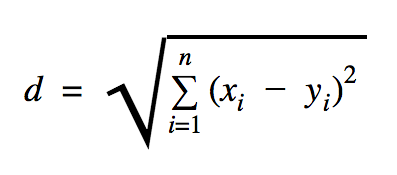In this formula, `n` is the number of dimensions of the space, `x` is the vector of the training data, and `y` is a new data point that we want to classify.

``````import os
import numpy as np
import tensorflow as tf

ccf_train_data = "train_dataset.csv"
ccf_test_data = "test_dataset.csv"

dataset_dir = os.path.abspath(os.path.join(os.path.dirname(__file__), '../datasets'))

ccf_train_filepath = os.path.join(dataset_dir, ccf_train_data)
ccf_test_filepath = os.path.join(dataset_dir, ccf_test_data)

from numpy import genfromtxt

data = []
labels = []

for d in csv_data:
data.append(d[:-1])
labels.append(d[-1])

return np.array(data), np.array(labels)

train_pl = tf.placeholder("float", [None, 28])
test_pl = tf.placeholder("float", )

pred = tf.argmin(knn_prediction, 0)

with tf.Session() as tf_session:
missed = 0

for i in xrange(len(test_dataset)):
knn_index = tf_session.run(pred, feed_dict={train_pl: train_dataset, test_pl: test_dataset[i]})

print "Predicted class {} -- True class {}".format(train_labels[knn_index], test_labels[i])

if train_labels[knn_index] != test_labels[i]:
missed += 1

tf.summary.FileWriter("../samples/article/logs", tf_session.graph)

print "Missed: {} -- Total: {}".format(missed, len(test_dataset))
``````

The dataset which we used in above example is one which can be found on the Kaggle datasets section. We used the one which contains transactions made by credit cards of European cardholders. We are using the data without any cleaning or filtering and as per the description in Kaggle for this dataset, it is highly unbalanced. The dataset contains 31 variables: Time, V1, …, V28, Amount, and Class. In this code sample we use only V1, …, V28 and Class. Class labels transactions which are fraudulent with 1 and those which aren’t with 0.

The code sample contains mostly the things which we described in previous sections with exception where we introduced the function for loading a dataset. The function `load_data(filepath)` will take a CSV file as an argument and will return a tuple with data and labels defined in CSV.

Just below that function, we have defined placeholders for the test and trained data. Trained data are used in the prediction model to resolve the labels for the input data that need to be classified. In our case, kNN use Euclidian distance to get the nearest label.

The error rate can be calculated by simple division with the number when a classifier missed by the total number of examples which in our case for this dataset is 0.2 (i.e., the classifier gives us the wrong data label for 20% of test data).

### Linear Regression

The linear regression algorithm looks for a linear relationship between two variables. If we label the dependent variable as y, and the independent variable as x, then we’re trying to estimate the parameters of the function `y = Wx + b`.

Linear regression is a widely used algorithm in the field of applied sciences. This algorithm allows adding in implementation two important concepts of machine learning: Cost function and the gradient descent method for finding the minimum of the function.

A machine learning algorithm that is implemented using this method must predict values of `y` as a function of `x` where a linear regression algorithm will determinate values `W` and `b`, which are actually unknowns and which are determined across training process. A cost function is chosen, and usually the mean square error is used where the gradient descent is the optimization algorithm used to find a local minimum of the cost function.

The gradient descent method is only a local function minimum, but it can be used in the search for a global minimum by randomly choosing a new start point once it has found a local minimum and repeating this process many times. If the number of minima of the function is limited and there are very high number of attempts, then there is a good chance that at some point the global minimum is spotted. Some more details about this technique we will leave for the article which we mentioned in the introduction section.

``````import tensorflow as tf
import numpy as np

test_data_size = 2000
iterations = 10000
learn_rate = 0.005

def generate_test_values():
train_x = []
train_y = []

for _ in xrange(test_data_size):
x1 = np.random.rand()
x2 = np.random.rand()
x3 = np.random.rand()
y_f = 2 * x1 + 3 * x2 + 7 * x3 + 4
train_x.append([x1, x2, x3])
train_y.append(y_f)

return np.array(train_x), np.transpose([train_y])

x = tf.placeholder(tf.float32, [None, 3], name="x")
W = tf.Variable(tf.zeros([3, 1]), name="W")
b = tf.Variable(tf.zeros(), name="b")
y = tf.placeholder(tf.float32, [None, 1])

cost = tf.reduce_mean(tf.square(y - model))

train_dataset, train_values = generate_test_values()

init = tf.global_variables_initializer()

with tf.Session() as session:
session.run(init)

for _ in xrange(iterations):

session.run(train, feed_dict={
x: train_dataset,
y: train_values
})

print "cost = {}".format(session.run(cost, feed_dict={
x: train_dataset,
y: train_values
}))

print "W = {}".format(session.run(W))
print "b = {}".format(session.run(b))
``````

Output:

``````cost = 3.1083032809e-05
W = [[ 1.99049103]
[ 2.9887135 ]
[ 6.98754263]]
b = [ 4.01742554]
``````

In the above example, we have two new variables, which we called `cost` and `train`. With those two variables, we defined an optimizer which we want to use in our training model and the function which we want to minimize.

At the end, the output parameters of `W` and `b` should be identical as those defined in the `generate_test_values` function. In line 17, we actually defined a function which we used to generate the linear data points to train where `w1=2`, `w2=3`, `w3=7` and `b=4`. Linear regression from the above example is multivariate where more than one independent variable are used.

## Conclusion

As you can see from this TensorFlow tutorial, TensorFlow is a powerful framework that makes working with mathematical expressions and multi-dimensional arrays a breeze—something fundamentally necessary in machine learning. It also abstracts away the complexities of executing the data graphs and scaling.

Over time, TensorFlow has grown in popularity and is now being used by developers for solving problems using deep learning methods for image recognition, video detection, text processing like sentiment analysis, etc. Like any other library, you may need some time to get used to the concepts that TensorFlow is built on. And, once you do, with the help of documentation and community support, representing problems as data graphs and solving them with TensorFlow can make machine learning at scale a less tedious process.

## Understanding the basics

### How are TensorFlow constants created?

In TensorFlow, constants are created using the constant function which takes a few parameters: Value, dtype (data type), shape, name and (verify_shape) shape verification.

### What is a TensorFlow session?

A session encapsulates the control and state of the TensorFlow runtime. A session without parameters will use the default graph created in the current session, otherwise the session class accepts a graph parameter, which is used in that session to be executed.

### What is TensorBoard?

TensorBoard is a visualization tool for analyzing data flow graphs. This can be useful for gaining better understanding of machine learning models.